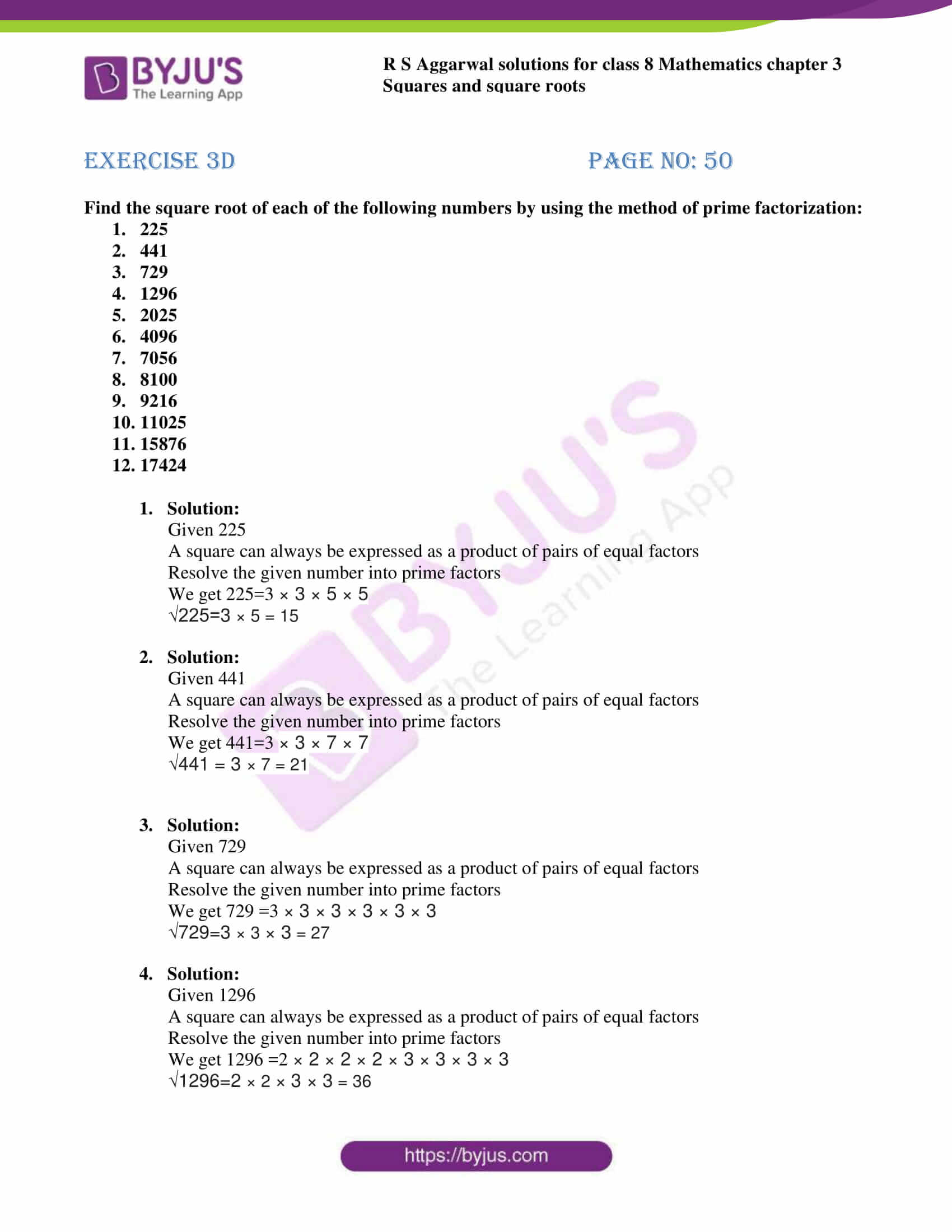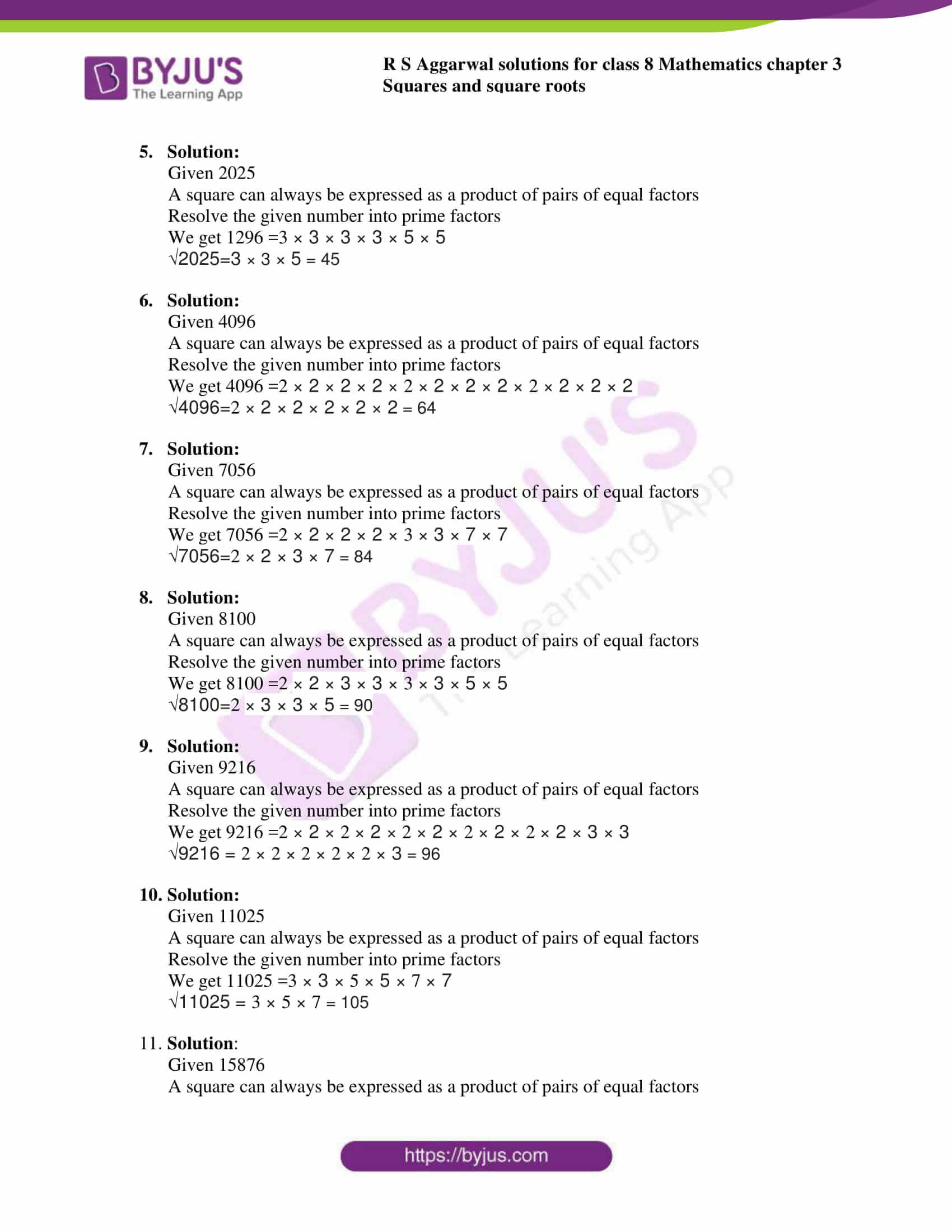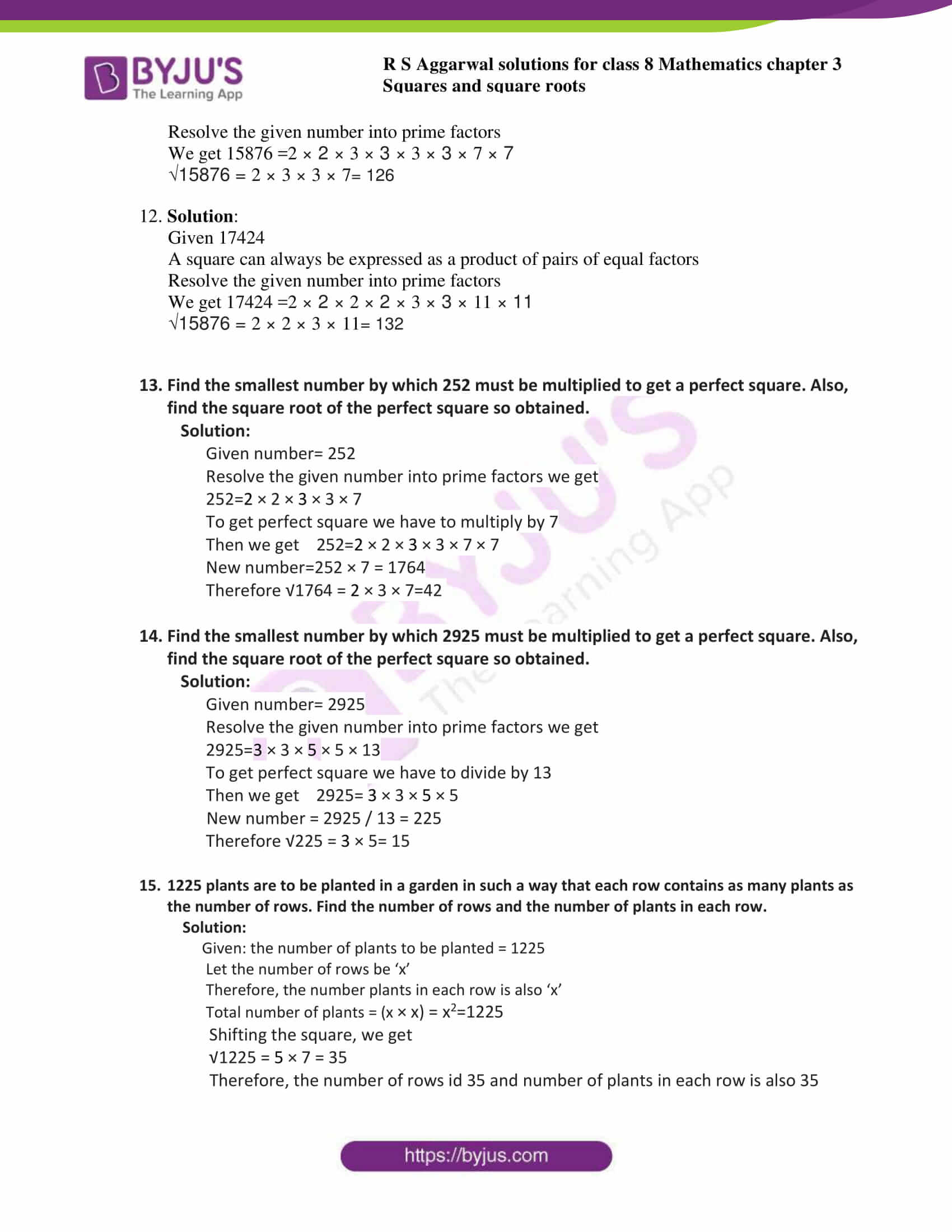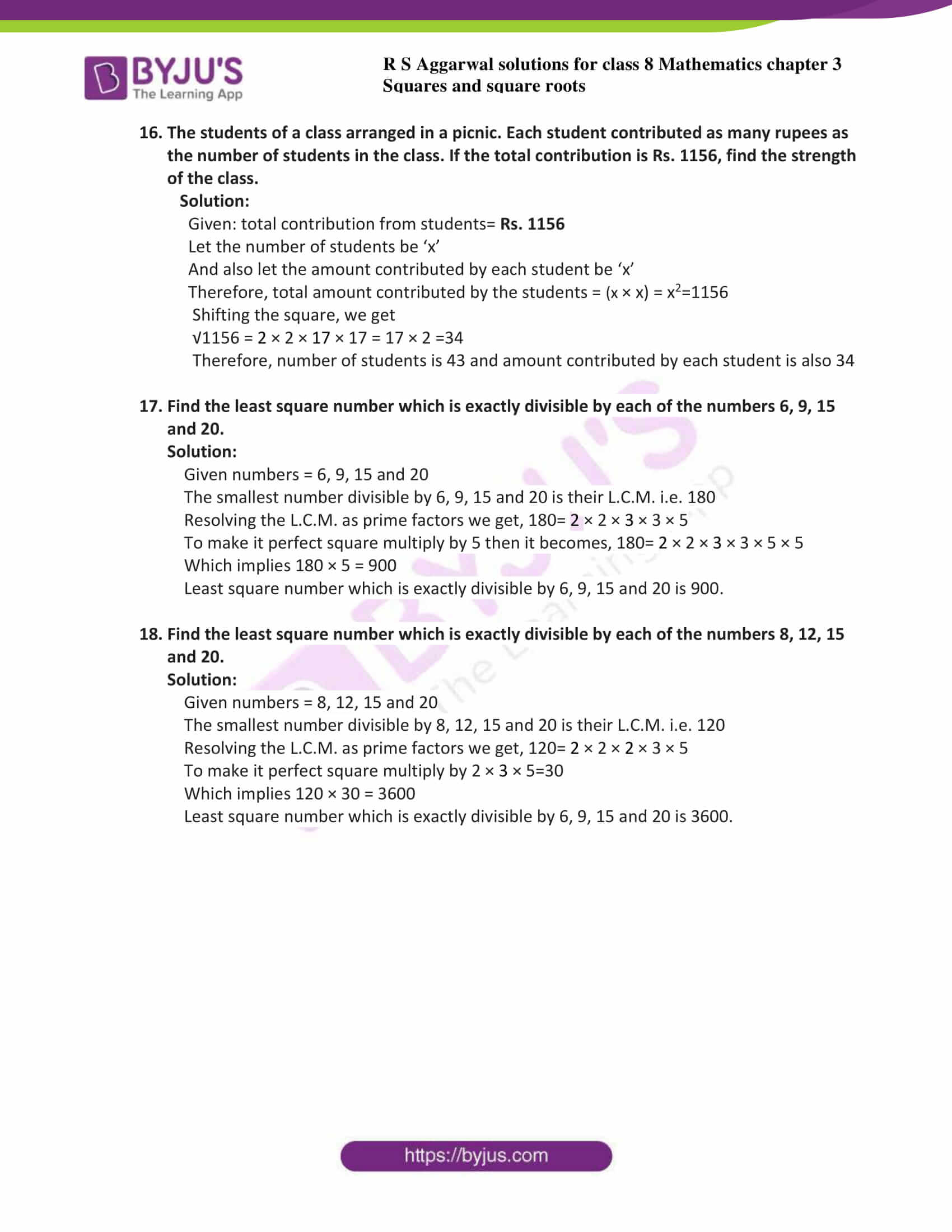# RS Aggarwal Solutions for Class 8 Maths Chapter 3 - Square and Square Roots Exercise 3D

RS Aggarwal Solutions for Class 8 Maths Chapter3 Exercise 3D are given below. BYJU’S experts have solved the questions present in the Exercise 3D in Maths, and this will help students in solving this exercise of Class 8 without any problems. Students who find it challenging to solve severe Math problems can refer to these RS Aggarwal 8th Maths solutions to get better guidance. Solving this exercise will assure that students excel in board exams. In this exercise, we shall study the concepts of square roots and finding a square root by prime factorisation method. By practising the RS Aggarwal Solutions for class 8, students will be able to grasp the concepts entirely, and they can attain good marks in their examinations.

## Download PDF of RS Aggarwal Solutions for Class 8 Maths Chapter 3 – Squares and Square Roots – Exercise 3D### Access answers to Maths RS Aggarwal Solutions for Class 8 Chapter 3 – Squares and Square Roots Exercise 3D

1. Find the square root of each of the following numbers by using the method of prime factorization:

1. 225
2. 441
3. 729
4. 1296
5. 2025
6. 4096
7. 7056
8. 8100
9. 9216
10. 11025
11. 15876
12. 17424
1. Solution:

Given 225

A square can always be expressed as a product of pairs of equal factors

Resolve the given number into prime factors

We get 225=3 × 3 × 5 × 5

√225=3 × 5 = 15

1. Solution:

Given 441

A square can always be expressed as a product of pairs of equal factors

Resolve the given number into prime factors

We get 441=3 × 3 × 7 × 7

√441 = 3 × 7 = 21

1. Solution:

Given 729

A square can always be expressed as a product of pairs of equal factors

Resolve the given number into prime factors

We get 729 =3 × 3 × 3 × 3 × 3 × 3

√729=3 × 3 × 3 = 27

1. Solution:

Given 1296

A square can always be expressed as a product of pairs of equal factors

Resolve the given number into prime factors

We get 1296 =2 × 2 × 2 × 2 × 3 × 3 × 3 × 3

√1296=2 × 2 × 3 × 3 = 36

1. Solution:

Given 2025

A square can always be expressed as a product of pairs of equal factors

Resolve the given number into prime factors

We get 1296 =3 × 3 × 3 × 3 × 5 × 5

√2025=3 × 3 × 5 = 45

1. Solution:

Given 4096

A square can always be expressed as a product of pairs of equal factors

Resolve the given number into prime factors

We get 4096 =2 × 2 × 2 × 2 × 2 × 2 × 2 × 2 × 2 × 2 × 2 × 2

√4096=2 × 2 × 2 × 2 × 2 × 2 = 64

1. Solution:

Given 7056

A square can always be expressed as a product of pairs of equal factors

Resolve the given number into prime factors

We get 7056 =2 × 2 × 2 × 2 × 3 × 3 × 7 × 7

√7056=2 × 2 × 3 × 7 = 84

1. Solution:

Given 8100

A square can always be expressed as a product of pairs of equal factors

Resolve the given number into prime factors

We get 8100 =2 × 2 × 3 × 3 × 3 × 3 × 5 × 5

√8100=2 × 3 × 3 × 5 = 90

1. Solution:

Given 9216

A square can always be expressed as a product of pairs of equal factors

Resolve the given number into prime factors

We get 9216 =2 × 2 × 2 × 2 × 2 × 2 × 2 × 2 × 2 × 2 × 3 × 3

√9216 = 2 × 2 × 2 × 2 × 2 × 3 = 96

1. Solution:

Given 11025

A square can always be expressed as a product of pairs of equal factors

Resolve the given number into prime factors

We get 11025 =3 × 3 × 5 × 5 × 7 × 7

√11025 = 3 × 5 × 7 = 105

1. Solution:

Given 15876

A square can always be expressed as a product of pairs of equal factors

Resolve the given number into prime factors

We get 15876 =2 × 2 × 3 × 3 × 3 × 3 × 7 × 7

√15876 = 2 × 3 × 3 × 7= 126

1. Solution:

Given 17424

A square can always be expressed as a product of pairs of equal factors

Resolve the given number into prime factors

We get 17424 =2 × 2 × 2 × 2 × 3 × 3 × 11 × 11

√15876 = 2 × 2 × 3 × 11= 132

1. Find the smallest number by which 252 must be multiplied to get a perfect square. Also, find the square root of the perfect square so obtained.

Solution:

Given number= 252

Resolve the given number into prime factors we get

252=2 × 2 × 3 × 3 × 7

To get perfect square we have to multiply by 7

Then we get 252=2 × 2 × 3 × 3 × 7 × 7

New number=252 × 7 = 1764

Therefore √1764 = 2 × 3 × 7=42

2. Find the smallest number by which 2925 must be multiplied to get a perfect square. Also, find the square root of the perfect square so obtained.

Solution:

Given number= 2925

Resolve the given number into prime factors we get

2925=3 × 3 × 5 × 5 × 13

To get perfect square we have to divide by 13

Then we get 2925= 3 × 3 × 5 × 5

New number = 2925 / 13 = 225

Therefore √225 = 3 × 5= 15

3. 1225 plants are to be planted in a garden in such a way that each row contains as many plants as the number of rows. Find the number of rows and the number of plants in each row.

Solution:

Given: the number of plants to be planted = 1225

Let the number of rows be ‘x’

Therefore, the number plants in each row is also ‘x’

Total number of plants = (x × x) = x2=1225

Shifting the square, we get

√1225 = 5 × 7 = 35

Therefore, the number of rows id 35 and number of plants in each row is also 35

4. The students of a class arranged in a picnic. Each student contributed as many rupees as the number of students in the class. If the total contribution is Rs. 1156, find the strength of the class.

Solution:

Given: total contribution from students= Rs. 1156

Let the number of students be ‘x’

And also let the amount contributed by each student be ‘x’

Therefore, total amount contributed by the students = (x × x) = x2=1156

Shifting the square, we get

√1156 = 2 × 2 × 17 × 17 = 17 × 2 =34

Therefore, number of students is 43 and amount contributed by each student is also 34

5. Find the least square number which is exactly divisible by each of the numbers 6, 9, 15 and 20.

Solution:

Given numbers = 6, 9, 15 and 20

The smallest number divisible by 6, 9, 15 and 20 is their L.C.M. i.e. 180

Resolving the L.C.M. as prime factors we get, 180= 2 × 2 × 3 × 3 × 5

To make it perfect square multiply by 5 then it becomes, 180= 2 × 2 × 3 × 3 × 5 × 5

Which implies 180 × 5 = 900

Least square number which is exactly divisible by 6, 9, 15 and 20 is 900.

6. Find the least square number which is exactly divisible by each of the numbers 8, 12, 15 and 20.

Solution:

Given numbers = 8, 12, 15 and 20

The smallest number divisible by 8, 12, 15 and 20 is their L.C.M. i.e. 120

Resolving the L.C.M. as prime factors we get, 120= 2 × 2 × 2 × 3 × 5

To make it perfect square multiply by 2 × 3 × 5=30

Which implies 120 × 30 = 3600

Least square number which is exactly divisible by 6, 9, 15 and 20 is 3600.

### Access other exercises of RS Aggarwal Solutions for Class 8 Maths Chapter 3 – Squares and Square Roots

Exercise 3A Solutions 6 questions

Exercise 3B Solutions 13 questions

Exercise 3C Solutions 8 questions

Exercise 3E Solutions 19 questions

Exercise 3F Solutions 12 questions

Exercise 3G Solutions 10 questions

Exercise 3H Solutions 19 questions

## RS Aggarwal Solutions for Class 8 Maths Chapter 3 – Squares and Square Roots Exercise 3D

Exercise 3D of RS Aggarwal Solutions for Chapter 3, Squares and Square Roots deals with the basic concepts related to square roots. We can say that this exercise mainly deals with the methods to find the square root of a perfect square by the prime factorisation method that the students have learned in this chapter. This exercise contains 18 questions based on the topics given below. Some of the topics focused prior to exercise 3D include the square root of a perfect square by the prime factorisation method.

The RS Aggarwal Solutions can help the students in practising and learning each and every concept as it provides solutions to all questions asked in the RS Aggarwal textbook. Those who aim to score high in the Maths of Class 8 are advised to practice all the questions present in RS Aggarwal as many times as possible. Hence, the preparation of exams using the RS Aggarwal solutions will definitely provide students with the advantage of scoring well.